# Proving Angles Congruent Quiz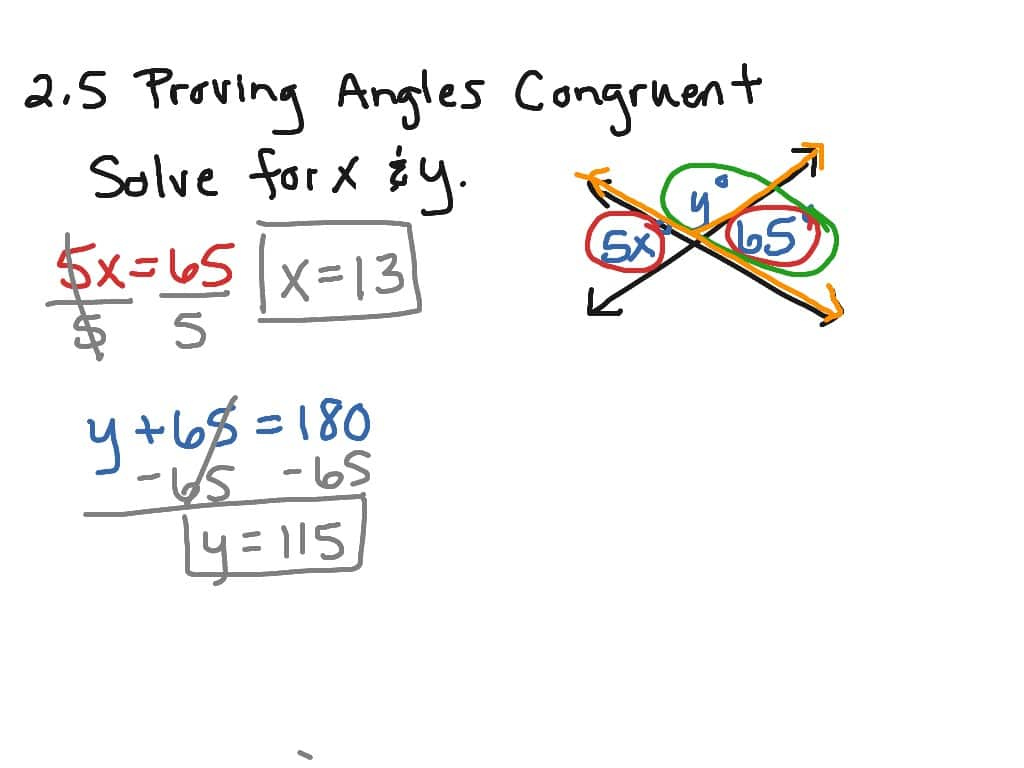We thoroughly check each answer to a question to provide you with the most correct answers. Found a mistake? Let us know about it through the REPORT button at the bottom of the page.

Proving Angles Congruent Quiz## Proving Angles Congruent Quiz Answers

1. Find  m\angle AEBm∠AEB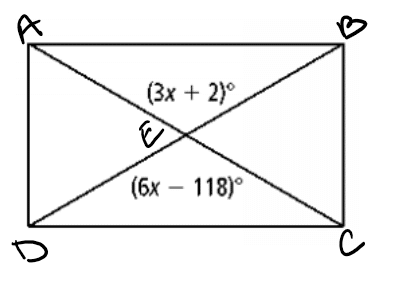• 40
• 28
• 120
• 122

2. What is the value of x?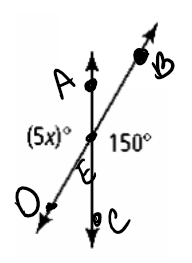• 150
• 30
• 145
• 15

3. Find  m\angle AEB.m∠AEB.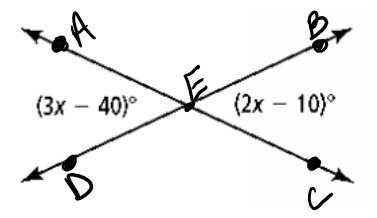• 150
• 30
• 130
• 50

4. Find ∠NOQ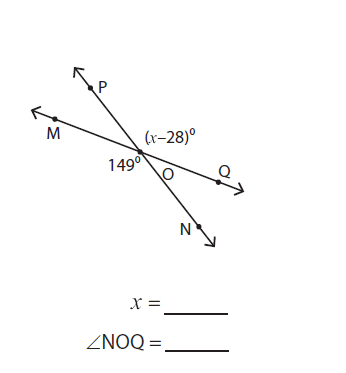• 31
• 59
• 180
• 149

5. ∠3 and ∠1 are _____________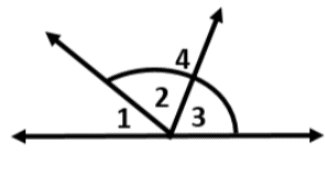• Nothing
• Vertical
• Complementary
• Supplementary

6. ∠1 and ∠2 are _____________• Complementary
• Supplementary
• Vertical

7. Find X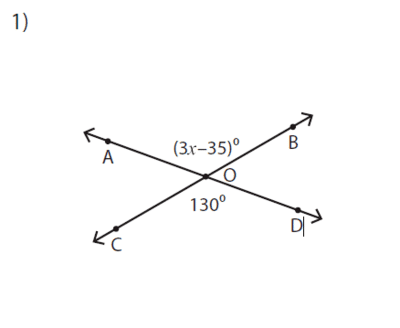• 28
• 5
• 55
• 40

8. Find ∠ AOC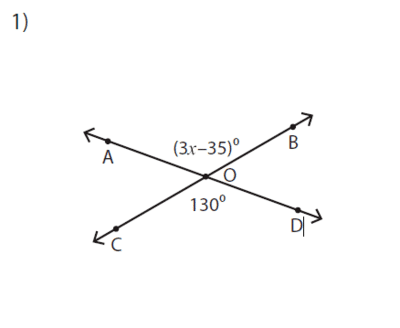• 130
• 50
• 40

9. ∠7 and ∠8 are _______________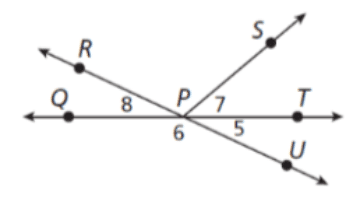• Vertical
• Nothing
• Supplementary

10. Find the missing angle.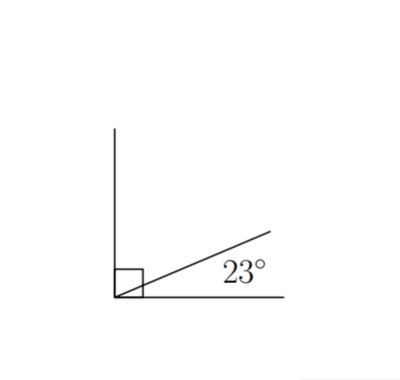• 43
• 47
• 57
• 67

11. Find the value of x.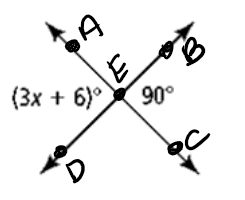• 28
• 30
• 32
• 90

12. Find  m\angle ECDm∠ECD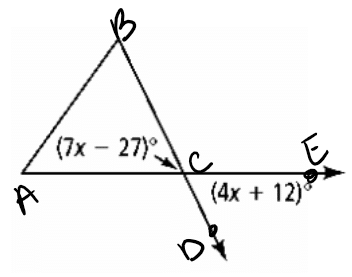• 13
• 64
• 90
• 180

13. Find  m\angle AEBm∠AEB• 40
• 28
• 120
• 122

14. Find the value of x.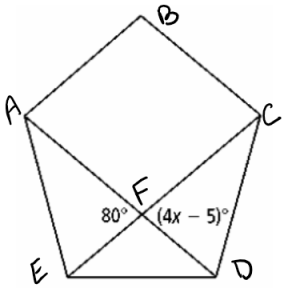• 18.75
• 21.25
• 80
• 158.75

15. Find the value of y.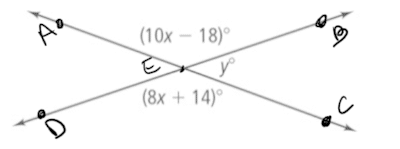• 16
• 32
• 38
• 142

16. Find  m\angle Am∠A  if  \angle A∠A  is congruent to its supplement,  \angle B.∠B.

• 45
• 90
• 180
• impossible to determine

17. Find  m\angle Am∠A  if  \angle A∠A  is twice as large as its complement,  \angle B.∠B.

• 30
• 45
• 60
• 90

18. Fill in the reason for number 3.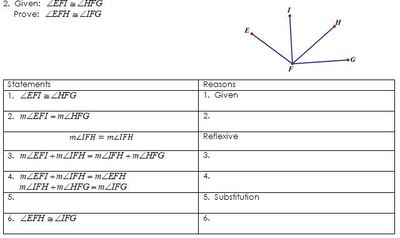• Angle Addition Postulate
• Definition of congruent angles
• Given

## Proving Angles Congruent Online Terms to Learn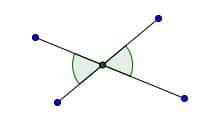Vertical Angles
2 angles whose sides form 2 pairs of opp. rays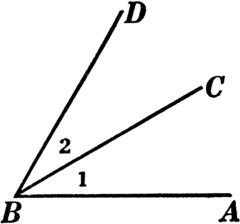2 coplanar angles w a common endpt and no common interior pts.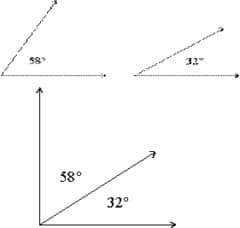Complementary angles
2 angles whose measurements have a sum of 90 degrees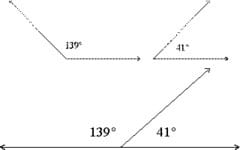Supplementary angles
2 angles whose measurements have a sum of 180 degrees.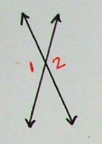Vertical angle theorem
Vertical angles are congruent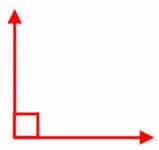Theorem 2-4
All right angles are congruent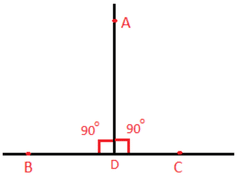Theorem 2-5
If 2 right angles are congruent and supplementary then each is a right angle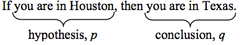Conditional Statement
if, then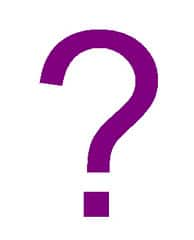Hypothesis
follows the “if” part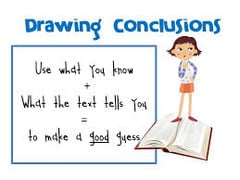Conclusion
follows the “then” part
truth value
the determination if the statement is true or false
converse

switches conclusion w hypothesis

if conclusion then hypothesisbiconditional

A true statement that combines a true conditional and a true converse. (if and only if)

hypothesis if and only if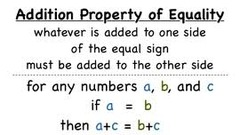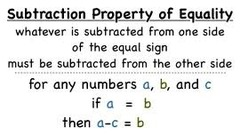subtraction POE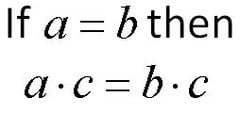multiplication POE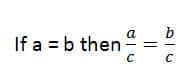division POE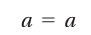reflexive POE
a = a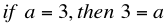symmetric POE
If a = b, then b = a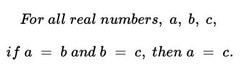transitive POE
If a = b and b = c, then a = c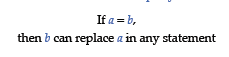substitution POE
if a=b, then “a” can replace “b” in an expression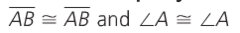reflexive POC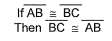symmetric POC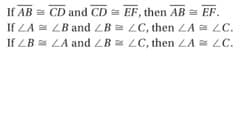transitive POC